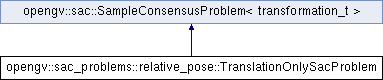OpenGV A library for solving calibrated central and non-central geometric vision problemsopengv::sac_problems::relative_pose::TranslationOnlySacProblem Class Reference

`#include <TranslationOnlySacProblem.hpp>`

Inheritance diagram for opengv::sac_problems::relative_pose::TranslationOnlySacProblem:## Public Types

typedef transformation_t model_tPublic Types inherited from opengv::sac::SampleConsensusProblem< transformation_t >
typedef transformation_t model_t

## Public Member Functions

Constructor. More...

Constructor. More...

virtual ~TranslationOnlySacProblem ()

virtual bool computeModelCoefficients (const std::vector< int > &indices, model_t &outModel) const
See parent-class.

virtual void getSelectedDistancesToModel (const model_t &model, const std::vector< int > &indices, std::vector< double > &scores) const
See parent-class.

virtual void optimizeModelCoefficients (const std::vector< int > &inliers, const model_t &model, model_t &optimized_model)
See parent-class.

virtual int getSampleSize () const
See parent-class.Public Member Functions inherited from opengv::sac::SampleConsensusProblem< transformation_t >
SampleConsensusProblem (bool randomSeed=true)
Contructor. More...

virtual ~SampleConsensusProblem ()
Destructor.

virtual void getSamples (int &iterations, std::vector< int > &samples)
Get samples for hypothesis generation. More...

virtual bool isSampleGood (const std::vector< int > &sample) const
Check if a set of samples for model generation is degenerate. More...

std::shared_ptr< std::vector< int > > getIndices () const
Get a pointer to the vector of indices used. More...

void drawIndexSample (std::vector< int > &sample)
Sub-function for getting samples for hypothesis generation. More...

virtual void getDistancesToModel (const model_t &model_coefficients, std::vector< double > &distances)
Compute the distances of all samples which respect to given model coefficients. More...

virtual void selectWithinDistance (const model_t &model_coefficients, const double threshold, std::vector< int > &inliers)
Select all the inlier samples whith respect to given model coefficients. More...

virtual int countWithinDistance (const model_t &model_coefficients, const double threshold)
Count all the inlier samples whith respect to given model coefficients. More...

void setIndices (const std::vector< int > &indices)
Set the indices_ variable (see member-description). More...

void setUniformIndices (int N)
Use this method if you want to use all samples. More...

int rnd ()
Get a random number. More...

## Protected AttributesPublic Attributes inherited from opengv::sac::SampleConsensusProblem< transformation_t >
int max_sample_checks_

std::shared_ptr< std::vector< int > > indices_

std::vector< int > shuffled_indices_

std::mt19937 rng_alg_
std-based random number generator algorithm.

std::shared_ptr< std::uniform_int_distribution<> > rng_dist_
std-based random number generator distribution.

std::shared_ptr< std::function< int()> > rng_gen_
std-based random number generator.

## Detailed Description

Functions for fitting a relative-pose model to a set of bearing-vector correspondences (using twopt). The viewpoints are assumed to be separated by translation only. Used within a random-sample paradigm for rejecting outlier correspondences.

## Member Typedef Documentation

The type of adapter that is expected by the methods

The model we are trying to fit (transformation)

## Constructor & Destructor Documentation

 opengv::sac_problems::relative_pose::TranslationOnlySacProblem::TranslationOnlySacProblem ( adapter_t & adapter, bool randomSeed = `true` )
inline

Constructor.

Parameters
 [in] adapter Visitor holding bearing vector correspondences etc. [in] randomSeed Whether to seed the random number generator with the current time.
 opengv::sac_problems::relative_pose::TranslationOnlySacProblem::TranslationOnlySacProblem ( adapter_t & adapter, const std::vector< int > & indices, bool randomSeed = `true` )
inline

Constructor.

Parameters
 [in] adapter Visitor holding bearing vector correspondences etc. [in] indices A vector of indices to be used from all available correspondences. [in] randomSeed Whether to seed the random number generator with the current time.
 virtual opengv::sac_problems::relative_pose::TranslationOnlySacProblem::~TranslationOnlySacProblem ( )
inlinevirtual

Destructor.